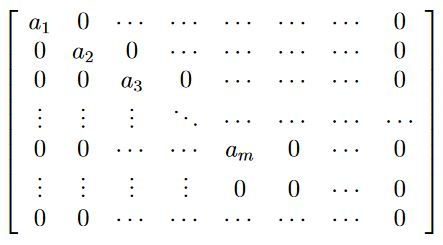# nLab Smith normal form

Contents

### Context

#### Algebra

higher algebra

universal algebra

# Contents

## Statement

###### Proposition

(matrices over principal ideal domains equivalent to Smith normal form)

For $R$ a commutative ring which is a principal ideal domain (for instance $R = \mathbb{Z}$ the integers), every matrix $A \in Mat_{n \times m}(R)$ with entries in $R$ is matrix equivalent to a diagonal matrix filled up with zeros:

There exist invertible matrices $P \in Mat_{n \times n}(R)$ and $Q \in Mat_{m \times m}(R)$ such that the product matrix $P A Q$ is of the following form:

$P A Q \;=\;$such that, moreover, each $a_i$ divides $a_{i+1}$.

The results is named after

• H.J.S. Smith, On systems of linear indeterminate equations and congruences Philos. Trans. Royal Soc. London cli, 293-326. Reprinted in The Collected Mathematical Papers of Henry John Stephen Smith, Volume 1. New York: Chelsea (1965)

Lecture notes include

• Patrick Morandi, The Smith Normal Form of a Matrix, 2005 (pdf)

• Sam Evans, Smith normal form over the integers (pdf)

• Bill Casselman, Hermite and Smith forms, 2011 (pdf)

• George Havas, Leon Sterling, Integer matrices and abelian groups (pdf, doi:10.1007/3-540-09519-5_94)

• George Havas, Bohdan Majewski, Integer matrices and diagonalization, J. Symbolic Computation (1997) 24, 399-408 (pdf)